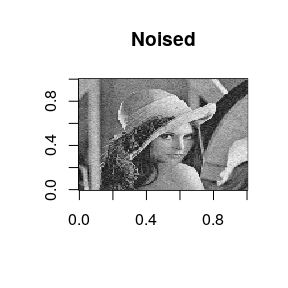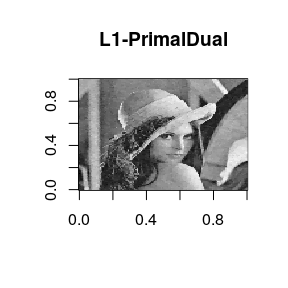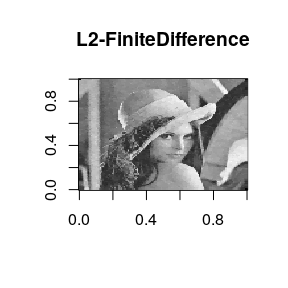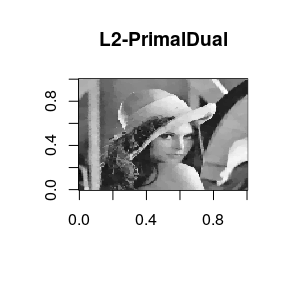# Denoising with tvR package

For a given noisy signal $$f$$, total variation regularization (also known as denoising) aims at recovering a cleaned version of signal $$u$$ by solving an equation of the following form $\min_u ~ E(u,f) + \lambda V(u)$ where $$E(u,f)$$ is a fidelity term that measures closeness of noisy signal $$f$$ to a desired solution $$u$$, and $$V(u)$$ a penalty term in pursuit of smoothness of a solution. For a differentiable function $$u : \Omega\rightarrow \mathbb{R}$$, total variation is defined as $V(u) = \int_{\Omega} \| \nabla u(x) \| dx$ and $$\lambda$$ a regularization parameter that balances fitness and smoothness defined by two terms.

Our tvR package provides two functions

• denoise1 for 1d signal (usually with time domain), and
• denoise2 for 2d signal such as image.

Let’s see two examples in the below.

library(tvR)
#> ------------------------------------------------------------
#> ** tvR
#> **  - Total Variation Regularization
#> ** Version    : 0.3.1      (2019)
#> ** Maintainer : Kisung You (kyoustat@gmail.com)
#> **
#> ** Please share any bugs or suggestions to the maintainer.
#> ------------------------------------------------------------

### Example : 1d signal with denoise1

We aim to solve TV-L2 problem, where $E(u,f) = \frac{1}{2} \int |u(x) - f(x)|^2 dx$ with a penalty $$V(u) = \sum_i |u_{i+1}-u_{i}|$$ with two algorithms, including 1) iterative clippling algorithm and 2) majorization-minorization method.

As an example, let’s create a stepped signal and add gaussian white noise with $$\sigma = 0.25$$

set.seed(1)
x = rep(sample(1:5,10,replace=TRUE), each=50) ## main signal
xnoised = x + rnorm(length(x), sd=0.25)       ## add noise

First, let’s compare how two algorithms perform with $$\lambda=1.0$$.

## apply denoising process
xproc1 = denoise1(xnoised, method = "TVL2.IC")
xproc2 = denoise1(xnoised, method = "TVL2.MM")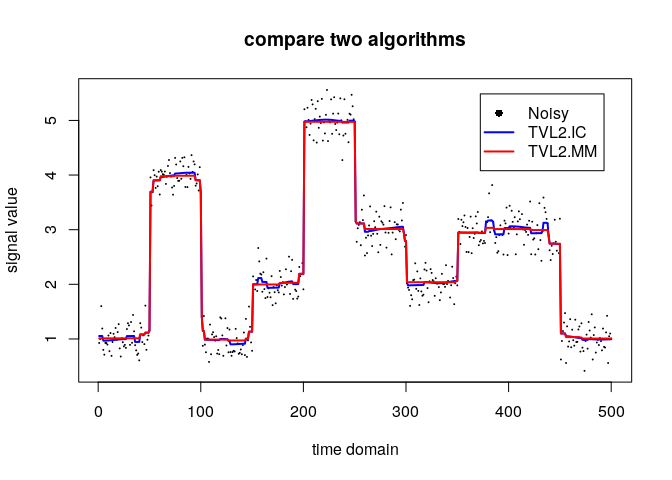which shows somewhat seemingly inconsistent results. However, this should be understood as induced by their internal algorithmic details such as stopping criterion. In such sense, let’s compare whether a single method is consistent with respect to the degree of regularization by varying parameters $$\lambda=10^{-3}, 10^{-2}, 10^{-1}, 1$$. For this comparison, we will use iterative clipping (TVL2.IC) algorithm.

compare = list()
for (i in 1:4){
compare[[i]] = denoise1(xnoised, lambda = 10^(i-4), method="TVL2.IC")
}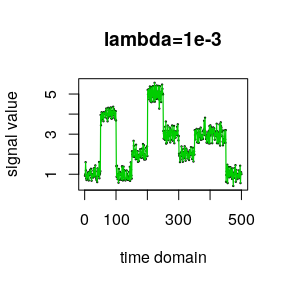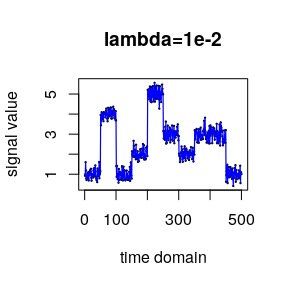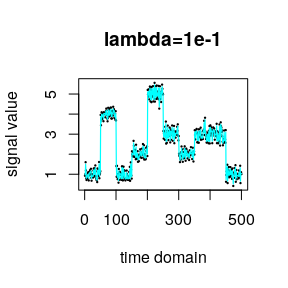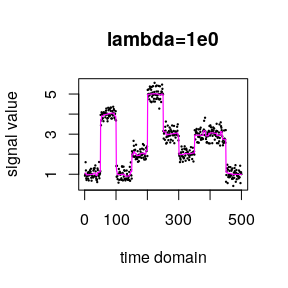An observation can be made that the larger the $$\lambda$$ is, the smoother the fitted solution becomes.

### Example : image denoising with denoise2

For a 2d signal case, we support both TV-L1 and TV-L2 problem, where $E_{L_1}(u,f) = \int_\Omega |u(x) - f(x)|_1 dx \\ E_{L_2}(u,f) = \int_\Omega |u(x) - f(x)|_2^2 dx$ given a 2-dimensional domain $$\Omega \subset \mathbb{R}^2$$ and a penalty $$V(u) = \sum (u_x^2 + u_y^2)^{1/2}$$. For TV-L1 problem, we provide primal-dual algorithm, whereas TV-L2 brings primal-dual algorithm as well as finite-difference scheme with fixed point iteration.

A typical yet major example of 2-dimensional signal is image, considering each pixel’s value as $$f(x,y)$$ at location $$(x,y)$$. We’ll use the gold standard image of Lena. In our example, we will use a version of gray-scale Lena image stored as a matrix of size $$128 \times 128$$ and add some gaussian noise as before with $$\sigma=10$$.

data(lena128)
xnoised <- lena128 + array(rnorm(128*128, sd=10), c(128,128))

Let’s see how different algorithms perform with $$\lambda=10$$.

## apply denoising process
xproc1 <- denoise2(xnoised, lambda=10, method="TVL1.PrimalDual")
xproc2 <- denoise2(xnoised, lambda=10, method="TVL2.FiniteDifference")
xproc3 <- denoise2(xnoised, lambda=10, method="TVL2.PrimalDual")